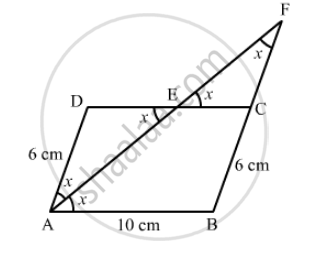Advertisement Remove all ads

# In a Parallelogram Abcd, Ab = 10 Cm, Ad = 6 Cm. the Bisector of ∠A Meets Dc in E, Aeand Bc Produced Meet at F. Find Te Length Cf. - Mathematics

In a parallelogram ABCDAB = 10 cm, AD = 6 cm. The bisector of ∠A meets DC in EAEand BC produced meet at F. Find te length CF

Advertisement Remove all ads

#### Solution$\text{ AE is the bisector of } \angle A .$
$\therefore \angle DAE = \angle BAE = x$
$\angle BAE = \angle AED = x (\text{ alternate angles })$
$\text{ Since opposite angles in ∆ ADE are equal, ∆ ADE is an isosceles triangle } .$
$\therefore AD = DE = 6 cm (\text{ sides opposite to equal angles })$
$AB = CD = 10 cm$
$CD = DE + EC$
$\Rightarrow EC = CD - DE$
$\Rightarrow EC = 10 - 6 = 4 cm$
$\angle DEA = \angle CEF = x (\text{ vertically opposite angle })$
$\angle EAD = \angle EFC = x (\text{ alternate angles })$
$\text{ Since opposite angles in } ∆ EFC \text{ are equal, ∆ EFC is an isosceles triangle } .$
$\therefore CF = CE = 4 \text{ cm (sides opposite to equal angles })$
$\therefore CF = 4\text{ cm }$

Is there an error in this question or solution?
Advertisement Remove all ads

#### APPEARS IN

RD Sharma Class 8 Maths
Chapter 17 Understanding Shapes-III (Special Types of Quadrilaterals)
Exercise 17.1 | Q 29 | Page 12
Advertisement Remove all ads

#### Video TutorialsVIEW ALL 

Advertisement Remove all ads
Share
Notifications

View all notifications

Forgot password?
Course